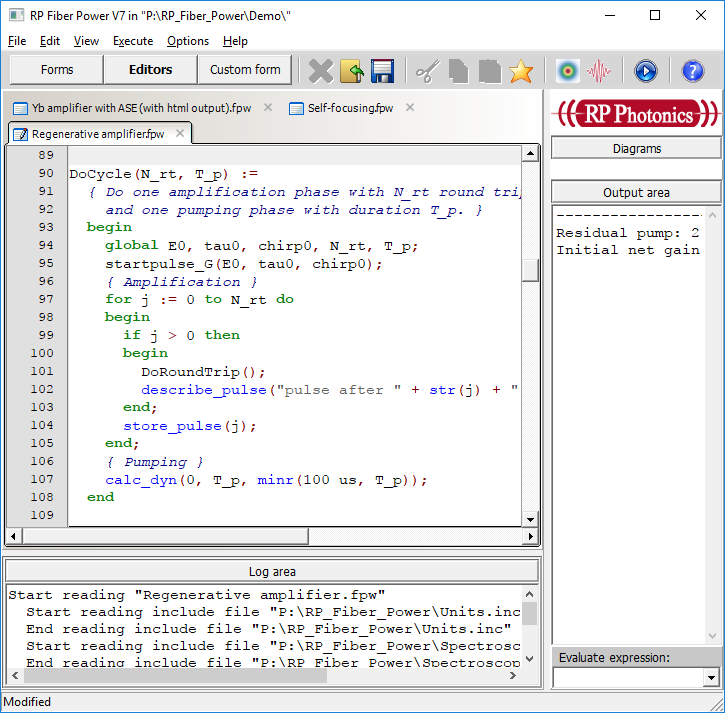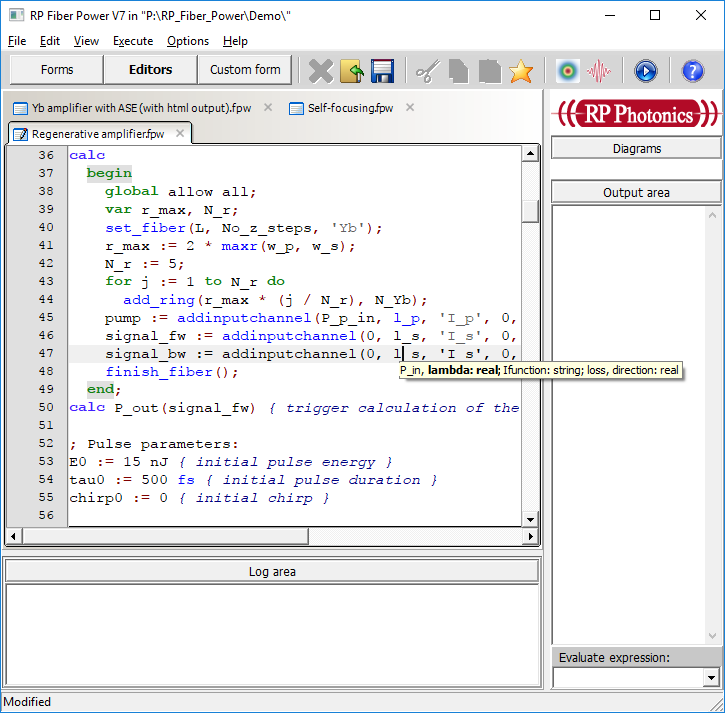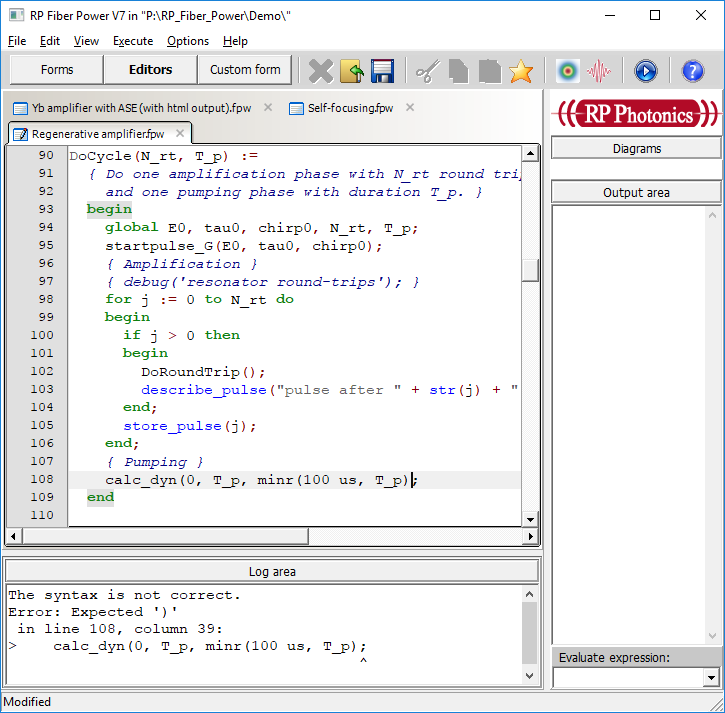## RP系列 | 编辑器功能 | 语法高亮显示、参数帮助、语法检查、自动代码重新格式化

2021-05-27 09:49发布```R_min:=150 mm { minimum curvature radius }
L_bend:=R_min*(90 deg)
show "L_bend: ", L_bend:d3:"m"
z1_bend:=(L_f-L_bend)/2
z2_bend:=(L_f+L_bend)/2
R(z):=
if z < z1_bend then
0
else if z < z2_bend then
R_min
else
0
theta(z):=
if z < z1_bend then
0
else if z < z2_bend then
-(z - z1_bend)/R_min
else
-pi/2```

```R_min := 150 mm { minimum curvature radius }
L_bend := R_min * (90 deg)
show "L_bend: ", L_bend:d3:"m"
z1_bend := (L_f - L_bend) / 2
z2_bend := (L_f + L_bend) / 2
R(z) :=
if z < z1_bend then
0
else if z < z2_bend then
R_min
else
0
theta(z) :=
if z < z1_bend then
0
else if z < z2_bend then
-(z - z1_bend) / R_min
else
-pi / 2```